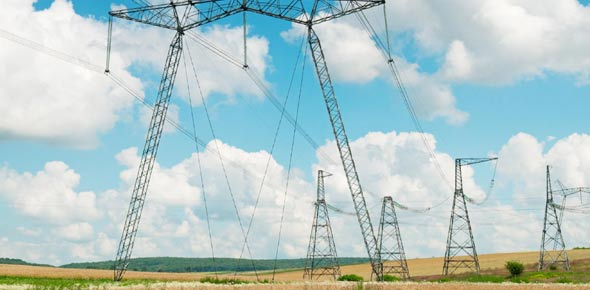# Electricity 2

14 QuestionsSettings.

Related Topics
• 1.
The image shows a potential difference-current graph which follows Ohm's law, we know this because the graph is linear, and has a constant slope.
• A.

True

• B.

False

• 2.
When is the potential difference not proportional to the voltage?
• A.

When the temperature remains constant

• B.

When the temperature differentiates

• C.

When the conductivity of the circuit remains constant

• D.

When the resistance of the circuit remains constant

• 3.
This one will require some thinking. Given that V = IR, we deduce that R = ___Further, if the slope of the graph is extremely low, then V/I, will be high, thus, we conclude that if the slope is low, resistance is ____, and if the slope is high, the resistance is ___
• A.

V/I, low, high

• B.

I/V, high, low

• C.

I/V, low, high

• D.

V/I, high, low

• 4.
The following is a graph of a light bulb. The derivative is decreasing, thus, we see that resistance______ as it gets hotter, this is due to more frequent collisions between electrons and metal atoms.
• A.

Increases

• B.

Decreases

• 5.
The following shows the graph of a diode. Once the potential difference reaches a _____ value, the component ______ Ohm's law, given that the temperature remains constant
• A.

Minimum, does not follow

• B.

Minimum, follows

• C.

Maximum, follows

• D.

Maximum, does not follow

• 6.
A diode completely resists current if the voltage is below a certain value, even if that value is negative
• A.

True

• B.

False

• 7.
Electricity can be dangerous, and cause electric shocks. What can these shocks do?
• A.

Interfere with nerve signals from brain to body

• B.

Cause heart attack

• C.

Cause muscle spasms

• D.

Cause breathing difficulties

• E.

Death

• F.

Overheated and faulty appliances

• G.

Electrical fires

• 8.
Check some precautions to make electricity safer
• A.

Use a fuse (short wire designed to melt and separate in event of excess current)

• B.

Earth wire (provide an alternative path if appliances develop faults)

• C.

Double insulation (plastic casing without wires connected to case, so case cannot become live)

• 9.
Energy is measured in ______
• 10.
What's the formula for energy?
• A.

E = VIt

• B.

E = VI

• 11.
1 Watt = 1 Joule per second --> 1 W = 1 J/s
• A.

True

• B.

False

• 12.
Power is measured in?
• 13.
What's the formula for power?
• A.

P = VIt

• B.

P = VI

• 14.
Select the advantages of placing bulbs in a parallel circuit
• A.

If one blows, the others with still run

• B.

Each branch is delivered the same voltage

• C.

You can place switches to control specific light bulbs